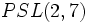# Subgroup structure of alternating group:A8

## Contents

View subgroup structure of particular groups | View other specific information about alternating group:A8

This article discusses the subgroup structure of alternating group:A8, which is the alternating group on the set$\{ 1, 2,3,4,5,6,7,8\}$. The group has order 20160.

## Family contexts

Family name Parameter values General information on subgroup structure of family
alternating group degree$n = 8$, i.e., the group$A_8$ subgroup structure of alternating groups
projective special linear group of degree four over a finite field field size$q = 2$, so field:F2, i.e., the group is$PSL(4,2)$. Note that because of isomorphism between linear groups over field:F2, this is also$SL(4,2)$,$PGL(4,2)$, and$GL(4,2)$.  ?

## Tables for quick information

FACTS TO CHECK AGAINST FOR SUBGROUP STRUCTURE: (finite group)
Lagrange's theorem (order of subgroup times index of subgroup equals order of whole group, so both divide it), |order of quotient group divides order of group (and equals index of corresponding normal subgroup)
Sylow subgroups exist, Sylow implies order-dominating, congruence condition on Sylow numbers|congruence condition on number of subgroups of given prime power order
normal Hall implies permutably complemented, Hall retract implies order-conjugate

### Quick summary

Item Value
Number of subgroups 48337
Compared with$A_n, n = 3,4,5,\dots$: 2, 10, 59, 501, 3786, 48337, ...
Number of conjugacy classes of subgroups 137
Compared with$A_n, n = 3,4,5,\dots$: 2, 5, 9, 22, 40, 137, ...
Number of automorphism classes of subgroups 112
Compared with$A_n, n = 3,4,5,\dots$: 2, 5, 9, 16, 37, 112, ...
Isomorphism classes of Sylow subgroups 2-Sylow: unitriangular matrix group:UT(4,2) (order 64).
3-Sylow: elementary abelian group:E9 (order 9).
5-Sylow: cyclic group:Z5 (order 5)
7-Sylow: cyclic group:Z7 (order 7)
Hall subgroups Other than the whole group, the trivial subgroup, and the Sylow subgroups, there are$\{ 2,3 \}$-Hall subgroups of order 576.
maximal subgroups maximal subgroups have orders 360, 576, 720, 1344, 2520
normal subgroups only the whole group and the trivial subgroup, because the group is simple. See alternating groups are simple.
subgroups that are simple non-abelian groups (other than the whole group) alternating group:A5 (order 60), projective special linear group:PSL(3,2) (order 168, the embedding arises via the natural permutation representation on the two-dimensional projective space over field:F2, which has size$2^2 + 2 + 1 = 7$, or via embedding it as$PSL(2,7)$ in the alternating group on a set of size$7 + 1 = 8$), alternating group:A6 (order 360), alternating group:A7 (order 2520)